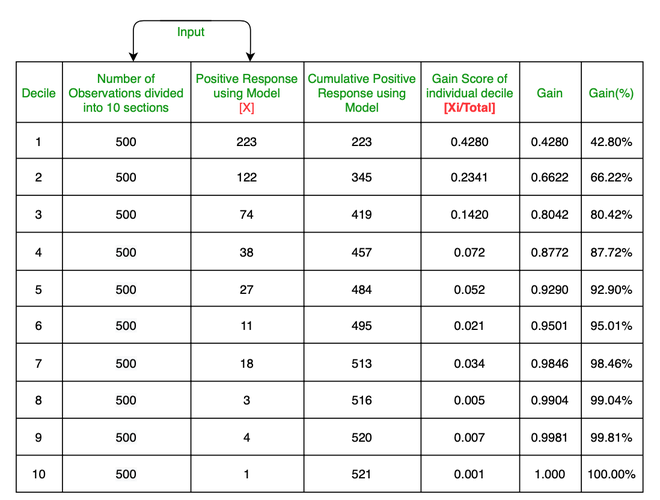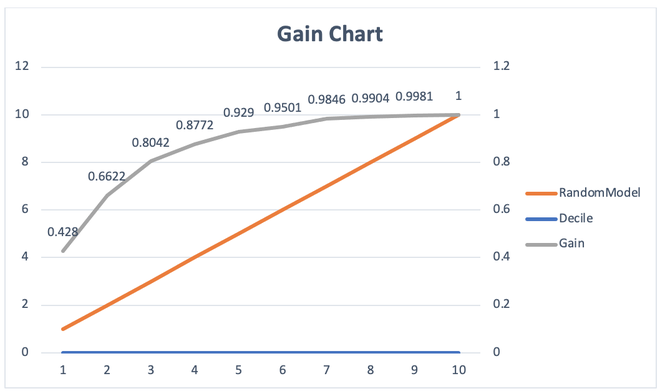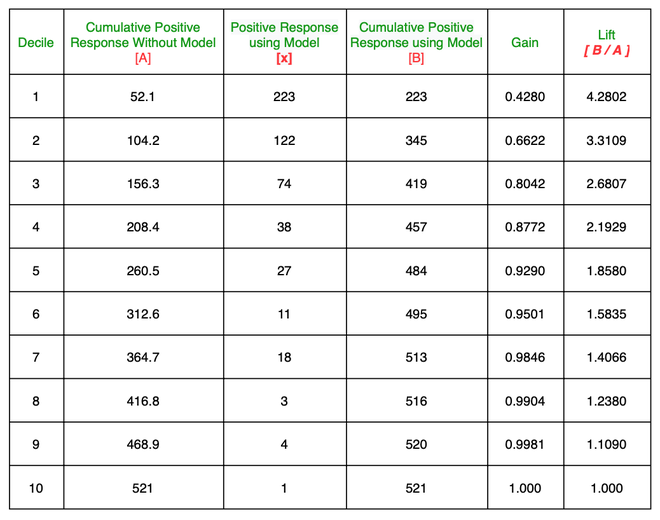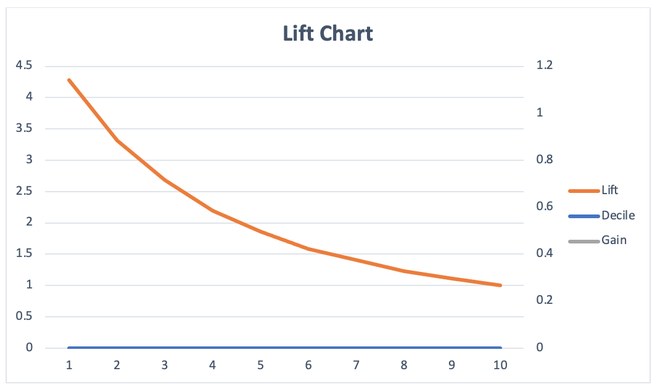# Understanding Gain Chart and Lift Chart

• Last Updated : 16 Mar, 2021

The gain chart and lift chart are two measures that are used for Measuring the benefits of using the model and are used in business contexts such as target marketing. It’s not just restricted to marketing analysis. It can also be used in other domains such as risk modeling, supply chain analytics, etc. In other words, Gain and Lift charts are two approaches used while solving classification problems with imbalanced data sets.

Example: In target marketing or marketing campaigns, the customer responses to campaigns are usually very low (in many cases the customers who respond to marketing campaigns are less than 1%). The organization will raise the cost for each customer contact and hence would like to minimize the cost of the marketing campaign and at the same time achieve desired response level from the customers.

The gain chart and lift chart is the measures in logistic regression that will help organizations to understand the benefits of using that model. So that better and more efficient output carry out.

The gain and lift chart is obtained using the following steps:

1. Predict the probability Y = 1 (positive) using the LR model and arrange the observation in the decreasing order of predicted probability [i.e., P(Y = 1)].
2. Divide the data sets into deciles. Calculate the number of positives (Y = 1) in each decile and the cumulative number of positives up to a decile.
3. Gain is the ratio between the cumulative number of positive observations up to a decile to the total number of positive observations in the data. The gain chart is a chart drawn between the gain on the vertical axis and the decile on the horizontal axis.4. Lift is the ratio of the number of positive observations up to decile i using the model to the expected number of positives up to that decile i based on a random model. Lift chart is the chart between the lift on the vertical axis and the corresponding decile on the horizontal axis.### Gain Chart Calculation:Ratio between the cumulative number of positive response up to a decile to the total number of positive responses in the data

#### Gain Chart:### Lift Chart Calculation:Ratio of the number of positive responses up to decile i using the model to the expected number of positives up to that decile i based on a random model

### Lift Chart:• Cumulative gains and lift charts are visual aids for measuring model performance.
• Both charts consist of Lift Curve (In Lift Chart) / Gain Chart (In Gain Chart) and Baseline (Blue Line for Lift, Orange Line for Gain).
• The Greater the area between the Lift / Gain and Baseline, the Better the model.
My Personal Notes arrow_drop_up